2019-11-16 12:01:56 weixin_44568780 阅读数 472
• ###### python数据分析与机器学习实战【2019新版】

购买课程后，请扫码进入学习群，获取唐宇迪老师答疑 Python数据分析与机器学习实战教程，该课程精心挑选真实的数据集为案例，通过python数据科学库numpy,pandas,matplot结合机器学习库scikit-learn完成一些列的机器学习案例。课程以实战为基础，所有课时都结合代码演示如何使用这些python库来完成一个真实的数据案例。算法与项目相结合，选择经典kaggle项目，从数据预处理开始一步步代码实战带大家入门机器学习。学完该课程即可： 1.掌握Python数据科学工具包，包括矩阵数据处理与可视化展示。 2.掌握机器学习算法原理推导，从数学上理解算法是怎么来的以及其中涉及的细节。 3.掌握每一个算法所涉及的参数，详解其中每一步对结果的影响。 4.熟练使用Python进行建模实战，基于真实数据集展开分析，一步步完成整个建模实战任务。

93869 人正在学习 去看看 唐宇迪

# 机器学习算法

1. 对于`分类算法`，通常我们输入大量`已分类数据`作为算法的`训练集``训练集``训练样本`的集合
2. 每个`训练样本`包含`特征`（也称`属性`）以及`目标变量`，在分类算法中，我们`目标变量`称为`类别`，并且假定分类问题只存在有限个数的类别
3. 机器学习算法目标变量的类型通常是`标称型`的如：男和女， 而在回归算法中通常是`连续型`
4. 特征或者属性通常是训练样本集的`列`，它们是独立测量得到的结果，多个特征联系在一 起共同组成一个训练样本。即每一训练样本可视为一个行向量，每一个特征即行向量的某一项，多个训练样本构成训练集矩阵，矩阵的相同列属于训练样本的同一特征

# k-近邻算法属于分类算法

## k-近邻算法的一般流程

1. 收集数据：可以使用任何方法。
2. 准备数据：距离计算所需要的数值，最好是结构化的数据格式。
3. 分析数据：可以使用任何方法。
4. 训练算法：此步骤不适用于k-近邻算法。
5. 测试算法：计算错误率。
6. 使用算法：首先需要输入样本数据和结构化的输出结果，然后运行k-近邻算法判定输入数据分别属于哪个分类，最后应用对计算出的分类执行后续的处理。
• 收集数据涉及爬虫，数据提取，数据处理等等各种方式
• 准备数据是将收集到的数据转化为计算机能够处理的`标准化格式`,如下图中的格式
•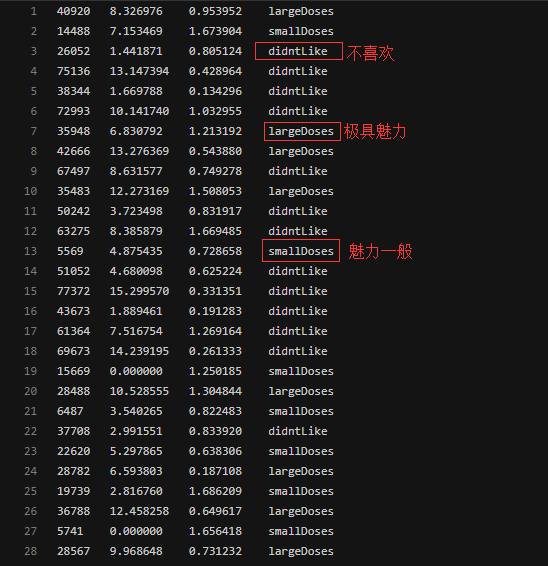- 分析数据的目的是保证数据的可用性，数据是否能正常用于分类区分，通常会用到`数据可视化`有关技术，如将高维数据转化为三维及以内数据进行可视化，比如使用`PCA主成分分析`进行最低信息损失的降维
• K-近邻算法不需要进行训练，每次使用都会调用所有训练数据
• 通过`测试集`对算法的分类成功率进行测试

## 算法原理：

1. K值的确定
2. 权重设置：即虽然最后选择了K个数据作为参考依据，但K个数据与测试数据的相对位置各不相同，因此需要对K个数据进行不同的权重设置
3. 距离的度量方式：计算距离是使用`欧氏距离`还是`马氏距离``巴氏距离``曼哈顿距离`等等，亦或有其他算法。

# 核心代码实现（本例为计算欧氏距离，权重均分）

``````inX -测试集，即分类对象
dataSet - 训练集
labes - 分类标签
k - 选择距离最小的k个点
sortedClassCount - 分类结果
``````

`inX`为一个行向量
`dataSet`为训练数据组成的numpy矩阵，每一行为一个训练数据行向量
`lables`为行向量，表征训练数据与测试数据的每一列代表特征

``````def classify0(inX, dataSet, labels, k):
#numpy函数shape返回dataSet的行数
dataSetSize = dataSet.shape
#在列向量方向上重复inX共1次(横向),行向量方向上重复inX共dataSetSize次(纵向)
diffMat = np.tile(inX, (dataSetSize, 1)) - dataSet
#二维特征相减后平方
sqDiffMat = diffMat**2
#sum()所有元素相加,sum(0)列相加,sum(1)行相加
sqDistances = sqDiffMat.sum(axis=1)
#开方,计算出距离
distances = sqDistances**0.5
#返回distances中元素从小到大排序后的索引值
sortedDistIndices = distances.argsort()
#定一个记录类别次数的字典
classCount = {}
for i in range(k):
#取出前k个元素的类别
voteIlabel = labels[sortedDistIndices[i]]
#dict.get(key,default=None),字典的get()方法,返回指定键的值,如果值不在字典中返回默认值。
#计算类别次数
classCount[voteIlabel] = classCount.get(voteIlabel,0) + 1
#python3中用items()替换python2中的iteritems()
#key=operator.itemgetter(1)根据字典的值进行排序
#key=operator.itemgetter(0)根据字典的键进行排序
#reverse降序排序字典
sortedClassCount = sorted(classCount.items(),key=operator.itemgetter(1),reverse=True)
print(sortedClassCount)
#返回次数最多的类别,即所要分类的类别
return sortedClassCount
``````

## `numpy.tile(mat,reps)`：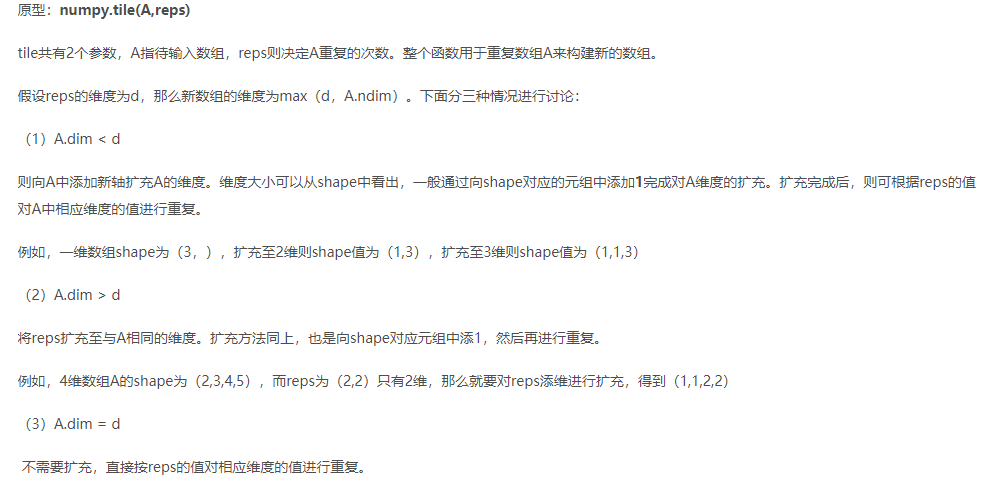``````>>> a
array([[1, 2],
[4, 5]])
>>> numpy.tile(a,(2,2))
array([[1, 2, 1, 2],
[4, 5, 4, 5],
[1, 2, 1, 2],
[4, 5, 4, 5]])
>>> numpy.tile(a,(2,2,2))
array([[[1, 2, 1, 2],
[4, 5, 4, 5],
[1, 2, 1, 2],
[4, 5, 4, 5]],

[[1, 2, 1, 2],
[4, 5, 4, 5],
[1, 2, 1, 2],
[4, 5, 4, 5]]])
>>> numpy.tile(a,(1,2,2))
array([[[1, 2, 1, 2],
[4, 5, 4, 5],
[1, 2, 1, 2],
[4, 5, 4, 5]]])
``````

# 海伦约会数据可视化代码

``````datingDataMat - 特征矩阵
datingLabels - 分类Label
``````
``````from matplotlib.font_manager import FontProperties
import matplotlib.lines as mlines
import matplotlib.pyplot as plt

def showdatas(datingDataMat, datingLabels):
#设置汉字格式
font = FontProperties(fname=r"c:\windows\fonts\simsunb.ttf", size=14)  ##需要查看自己的电脑是否会包含该字体
#将fig画布分隔成1行1列,不共享x轴和y轴,fig画布的大小为(13,8)
#当nrow=2,nclos=2时,代表fig画布被分为四个区域,axs表示第一行第一个区域
fig, axs = plt.subplots(nrows=2, ncols=2,sharex=False, sharey=False, figsize=(13,8))

numberOfLabels = len(datingLabels)
LabelsColors = []
for i in datingLabels:
if i == 1:
LabelsColors.append('black')
if i == 2:
LabelsColors.append('orange')
if i == 3:
LabelsColors.append('red')
#画出散点图,以datingDataMat矩阵的第一(飞行常客例程)、第二列(玩游戏)数据画散点数据,散点大小为15,透明度为0.5
axs.scatter(x=datingDataMat[:,0], y=datingDataMat[:,1], color=LabelsColors,s=15, alpha=.5)
#设置标题,x轴label,y轴label
axs0_title_text = axs.set_title(u'每年获得的飞行常客里程数与玩视频游戏所消耗时间占比',FontProperties=font)
axs0_xlabel_text = axs.set_xlabel(u'每年获得的飞行常客里程数',FontProperties=font)
axs0_ylabel_text = axs.set_ylabel(u'玩视频游戏所消耗时间占比',FontProperties=font)
plt.setp(axs0_title_text, size=9, weight='bold', color='red')
plt.setp(axs0_xlabel_text, size=7, weight='bold', color='black')
plt.setp(axs0_ylabel_text, size=7, weight='bold', color='black')

#画出散点图,以datingDataMat矩阵的第一(飞行常客例程)、第三列(冰激凌)数据画散点数据,散点大小为15,透明度为0.5
axs.scatter(x=datingDataMat[:,0], y=datingDataMat[:,2], color=LabelsColors,s=15, alpha=.5)
#设置标题,x轴label,y轴label
axs1_title_text = axs.set_title(u'每年获得的飞行常客里程数与每周消费的冰激淋公升数',FontProperties=font)
axs1_xlabel_text = axs.set_xlabel(u'每年获得的飞行常客里程数',FontProperties=font)
axs1_ylabel_text = axs.set_ylabel(u'每周消费的冰激淋公升数',FontProperties=font)
plt.setp(axs1_title_text, size=9, weight='bold', color='red')
plt.setp(axs1_xlabel_text, size=7, weight='bold', color='black')
plt.setp(axs1_ylabel_text, size=7, weight='bold', color='black')

#画出散点图,以datingDataMat矩阵的第二(玩游戏)、第三列(冰激凌)数据画散点数据,散点大小为15,透明度为0.5
axs.scatter(x=datingDataMat[:,1], y=datingDataMat[:,2], color=LabelsColors,s=15, alpha=.5)
#设置标题,x轴label,y轴label
axs2_title_text = axs.set_title(u'玩视频游戏所消耗时间占比与每周消费的冰激淋公升数',FontProperties=font)
axs2_xlabel_text = axs.set_xlabel(u'玩视频游戏所消耗时间占比',FontProperties=font)
axs2_ylabel_text = axs.set_ylabel(u'每周消费的冰激淋公升数',FontProperties=font)
plt.setp(axs2_title_text, size=9, weight='bold', color='red')
plt.setp(axs2_xlabel_text, size=7, weight='bold', color='black')
plt.setp(axs2_ylabel_text, size=7, weight='bold', color='black')
#设置图例
didntLike = mlines.Line2D([], [], color='black', marker='.',
markersize=6, label='didntLike')
smallDoses = mlines.Line2D([], [], color='orange', marker='.',
markersize=6, label='smallDoses')
largeDoses = mlines.Line2D([], [], color='red', marker='.',
markersize=6, label='largeDoses')
#添加图例
axs.legend(handles=[didntLike,smallDoses,largeDoses])
axs.legend(handles=[didntLike,smallDoses,largeDoses])
axs.legend(handles=[didntLike,smallDoses,largeDoses])
#显示图片
plt.show()
``````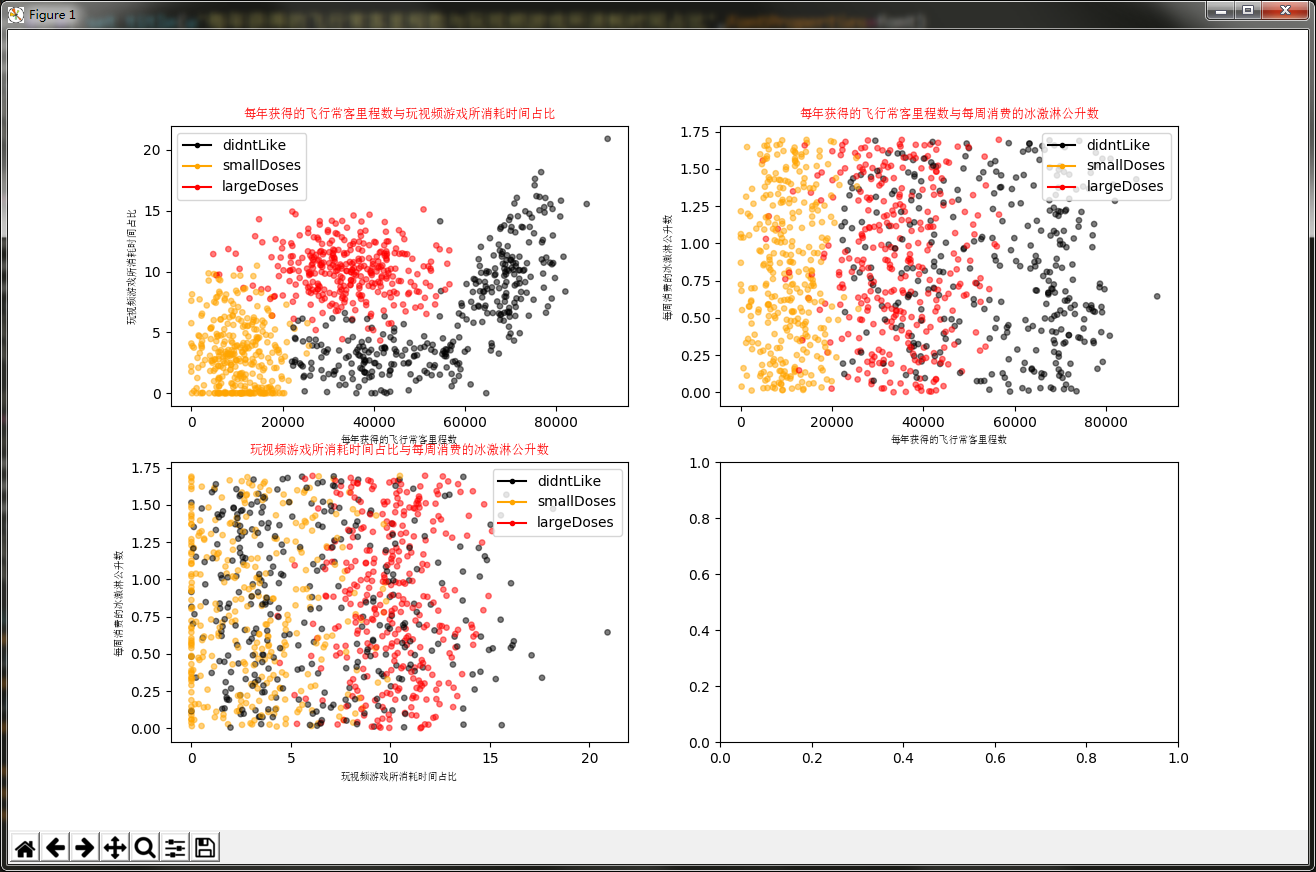# 手写数字识别与通过Sklearn实现调用KNN算法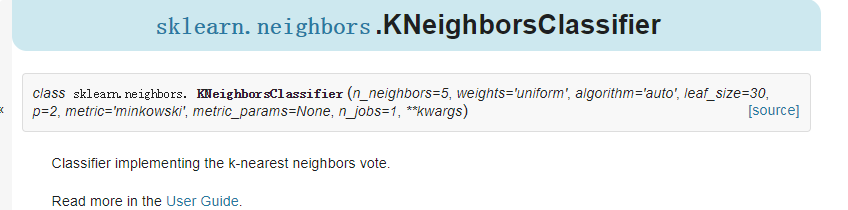`sklearn.neighbors.KNeighborsClassifier`函数一共有8个参数

## 最简单的例子用下面三个

``````from sklearn.neighbors import KNeighborsClassifier as kNN

#构建kNN分类器
neigh = kNN(n_neighbors = 3, algorithm = 'auto')
#拟合模型, trainingMat为训练矩阵,hwLabels为对应的标签
neigh.fit(trainingMat, hwLabels)
classifierResult = neigh.predict(vectorUnderTest)
``````

## SKlearn实现完整代码

``````# -*- coding: UTF-8 -*-
import numpy as np
import operator
from os import listdir
from sklearn.neighbors import KNeighborsClassifier as kNN

"""

Parameters:
filename - 文件名
Returns:
returnVect - 返回的二进制图像的1x1024向量

Modify:
2017-07-15
"""
def img2vector(filename):
#创建1x1024零向量
returnVect = np.zeros((1, 1024))
#打开文件
fr = open(filename)
#按行读取
for i in range(32):
#读一行数据
#每一行的前32个元素依次添加到returnVect中
for j in range(32):
returnVect[0, 32*i+j] = int(lineStr[j])
#返回转换后的1x1024向量
return returnVect

"""

Parameters:
无
Returns:
无

Modify:
2017-07-15
"""
def handwritingClassTest():
#测试集的Labels
hwLabels = []
#返回trainingDigits目录下的文件名
trainingFileList = listdir('trainingDigits')
#返回文件夹下文件的个数
m = len(trainingFileList)
#初始化训练的Mat矩阵,测试集
trainingMat = np.zeros((m, 1024))
#从文件名中解析出训练集的类别
for i in range(m):
#获得文件的名字
fileNameStr = trainingFileList[i]
#获得分类的数字
classNumber = int(fileNameStr.split('_'))
#将获得的类别添加到hwLabels中
hwLabels.append(classNumber)
#将每一个文件的1x1024数据存储到trainingMat矩阵中
trainingMat[i,:] = img2vector('trainingDigits/%s' % (fileNameStr))
#构建kNN分类器
neigh = kNN(n_neighbors = 3, algorithm = 'auto')
#拟合模型, trainingMat为训练矩阵,hwLabels为对应的标签
neigh.fit(trainingMat, hwLabels)
#返回testDigits目录下的文件列表
testFileList = listdir('testDigits')
#错误检测计数
errorCount = 0.0
#测试数据的数量
mTest = len(testFileList)
#从文件中解析出测试集的类别并进行分类测试
for i in range(mTest):
#获得文件的名字
fileNameStr = testFileList[i]
#获得分类的数字
classNumber = int(fileNameStr.split('_'))
#获得测试集的1x1024向量,用于训练
vectorUnderTest = img2vector('testDigits/%s' % (fileNameStr))
#获得预测结果
# classifierResult = classify0(vectorUnderTest, trainingMat, hwLabels, 3)
classifierResult = neigh.predict(vectorUnderTest)
print("分类返回结果为%d\t真实结果为%d" % (classifierResult, classNumber))
if(classifierResult != classNumber):
errorCount += 1.0
print("总共错了%d个数据\n错误率为%f%%" % (errorCount, errorCount/mTest * 100))

"""

Parameters:
无
Returns:
无

Modify:
2017-07-15
"""
if __name__ == '__main__':
handwritingClassTest()

``````

k算法 机器学习实战 相关内容

2019-09-24 20:51:07 weixin_40264772 阅读数 20
• ###### python数据分析与机器学习实战【2019新版】

购买课程后，请扫码进入学习群，获取唐宇迪老师答疑 Python数据分析与机器学习实战教程，该课程精心挑选真实的数据集为案例，通过python数据科学库numpy,pandas,matplot结合机器学习库scikit-learn完成一些列的机器学习案例。课程以实战为基础，所有课时都结合代码演示如何使用这些python库来完成一个真实的数据案例。算法与项目相结合，选择经典kaggle项目，从数据预处理开始一步步代码实战带大家入门机器学习。学完该课程即可： 1.掌握Python数据科学工具包，包括矩阵数据处理与可视化展示。 2.掌握机器学习算法原理推导，从数学上理解算法是怎么来的以及其中涉及的细节。 3.掌握每一个算法所涉及的参数，详解其中每一步对结果的影响。 4.熟练使用Python进行建模实战，基于真实数据集展开分析，一步步完成整个建模实战任务。

93869 人正在学习 去看看 唐宇迪

## 机器学习实战-K近邻算法（分类电影）

``````#-*- Coding:utf-8 -*-
# Author:LHF  Time:2019/9/21
from numpy import *
import operator  #运算符模块

def creatDataSet():
group = array([[1.0,101],[5,89],[108,5],[115,8]])
labels = ['爱情片','爱情片','动作片','动作片']
return group,labels

def classify0(inx,dataSet,labels,k):
dateSetSize = dataSet.shape#numpy函数shape返回dataSet的行数
diffMat = tile(inx,(dateSetSize,1)) - dataSet #在行向量上inx有dataSetSize次，在列向量上inx有一次
sqDiffMat = diffMat ** 2#二维矩阵相减后每个数据求平方
sqDistances = sqDiffMat.sum(axis = 1)#求出每一行数据的总和
distances = sqDistances ** 0.5#将每一行数据的总和开平方
sortedDistIndicies = distances.argsort()#按照distances中数据的大小排序，然后替换成在原来每行数据的索引
classCount = {}
for i in range(k):#多数表决法，按大小顺序，求出前k个
voteIlabel = labels[sortedDistIndicies[i]]
classCount[voteIlabel] = classCount.get(voteIlabel,0) + 1#get(指定键，默认值) get返回指定键的值，如果没有，返回默认值
#key = operator.itemgetter(1) 根据字典的值进行排序
# key = operator.itemgetter(0) 根据字典的键进行排序
sortedClassCount = sorted(classCount.items(),key = operator.itemgetter(1),reverse = True)
#拿出字典中第一个键值对的键
return sortedClassCount

if __name__ == '__main__':
group,labels = creatDataSet()
test = [101,20]
test_class = classify0(test,group,labels,3)
print(test_class)
``````

**

## 运行结果

**

``````动作片
``````

k算法 机器学习实战 相关内容

2017-11-09 23:01:41 Im_Chenxi 阅读数 380
• ###### python数据分析与机器学习实战【2019新版】

购买课程后，请扫码进入学习群，获取唐宇迪老师答疑 Python数据分析与机器学习实战教程，该课程精心挑选真实的数据集为案例，通过python数据科学库numpy,pandas,matplot结合机器学习库scikit-learn完成一些列的机器学习案例。课程以实战为基础，所有课时都结合代码演示如何使用这些python库来完成一个真实的数据案例。算法与项目相结合，选择经典kaggle项目，从数据预处理开始一步步代码实战带大家入门机器学习。学完该课程即可： 1.掌握Python数据科学工具包，包括矩阵数据处理与可视化展示。 2.掌握机器学习算法原理推导，从数学上理解算法是怎么来的以及其中涉及的细节。 3.掌握每一个算法所涉及的参数，详解其中每一步对结果的影响。 4.熟练使用Python进行建模实战，基于真实数据集展开分析，一步步完成整个建模实战任务。

93869 人正在学习 去看看 唐宇迪

# 内容

1.算法简介......对KNN算法有一个具体的模型概念

2.代码实现......对机器学习实战书中约会网站实例的代码实现

3.算法扩展......参数选择及kd树

k-近邻算法的一般流程：

# 2.代码实现

## 2.1 文本转换

```def file2matrix(filename):
fr = open(filename)
numberOflines = len(arrayOnlines)#得到文件行数
returnMat = zeros((numberOflines,3))#创建numpy矩阵
classLableVector = []#定义其为一个列表
index = 0
#解析文件数据到列表
for line in arrayOnlines:
line = line.strip()#根据()里的参数分割内容，默认是空格
listFromLine = line.split('\t')#返回分割后的字符串列表。
returnMat[index,:] = listFromLine[0:3]#将文件的内容矩阵化，并读取前三个内容
classLableVector.append(int(listFromLine[-1]))#读取最后一个内容，必须明确告诉解释器，列表中存储的元素为整形，否则会当作字符串进行处理
index += 1
return returnMat,classLableVector```

测试时和预测时输入的是一个1*N的矩阵
标签Lables是一个M*1的矩阵

## 2.2归一化数值每年获得的飞行常客里程数的影响远远大于其他两项，在处理这种不同范围的特征值时，我们通常采用的方法是将数值进行归一化。

```#归一化处理
def autoNorm(dataSet):#参数为输入的特征值矩阵
minVals = dataSet.min(0)#找出每一特征值的最小值
maxVals = dataSet.max(0)#找出每一特征值的最大值
ranges = maxVals - minVals
normDataSet = zeros(shape(dataSet))#初始化尺寸归一化后的矩阵
m = dataSet.shape#行数即样本个数
normDataSet = dataSet - tile(minVals, (m,1))#对应公式的 (oldValue - min)
normDataSet = normDataSet/tile(ranges, (m,1))#可直接相除，相当于每个元素除以对应元素
return normDataSet, ranges, minVals```## 2.3分类器

(1) 计算已知类别数据集中的点与当前点的之间距离

(2) 按照距离递增次序排序

(3) 选取与当前点距离最小的k个点

(4) 确定前k个点所在的类别的出现频率

(5) 返回前k个点出现频率最高的类别作为当前点的预测分类根据伪代码写出代码：

```def classify0(inX, dataSet, lables, k):#定义一个分类器
dataSetSize = dataSet.shape #得到训练集样本的个数
diffMat = tile(inX, (dataSetSize,1)) - dataSet #将输入向量X进行扩展成训练集的行数，并减去训练集中的值 进行差值运算
#进行欧式距离的运算
sqDiffMat = diffMat ** 2
sqDistances = sqDiffMat.sum(axis=1)#axis=1 是将矩阵或数组的行进行相加
distances = sqDistances ** 0.5
sortedDistIndicies = distances.argsort()
#argsort()按照一定的大小顺序进行排列 默认是按照升序进行排列
classCount = {}#字典类型
#对最近的k个训练集中的实例进行标签的计数工作
for i in range(k):
voteIlabel = lables[sortedDistIndicies[i]]
classCount[voteIlabel] = classCount.get(voteIlabel,0) + 1
sortedClassCount = sorted(classCount.items(), key=operator.itemgetter(1), reverse=True)#按每个标签对应的数量升序进行排列
return sortedClassCount#从k个标签数中最多的那个标签作为输入x的预估值```

(1).四个参数，前三个在前面也表述是三个矩阵，而参数k由定义知是选择的距离最近的k个点，机器学习实战选择了3，关于k的具体在第四部分在叙述。
(2).shape 函数  shape返回的是行数，shape返回的是列数，shape是返回行数列数的一个数组。
(3).argsort()函数 按照一定的大小顺序进行排列，默认是按照升序进行排列
(4).在倒数第二行的代码中，我第一次按照书中代码执行发现会报错   AttributeError: 'dict' object has no attribute 'iteritems'    然后发现代码没有问题，分析报错原因和iteritems这个迭代器有关，而且自己学python时不记得有用到这个(我学的书用的python3)，就觉得是不是python2和3的差，就去百度了下，发现又一个博客下写到：
Python 3.x里面，iteritems()和viewitems()这两个方法都已经删除，可用item()替代，于是我就尝试用items()替代，发现没有了错误，而且功能成功实现。

## 2.4测试程序

```def datingClassTest():
hoRitio = 0.1
datingDataMat, datingLabels = file2matrix('datingTestSet.txt')
normMat, ranges, minVals = autoNorm(datingDataMat)
m = normMat.shape
numTestVecs = int(m*hoRitio)
errorCount = 0.0
for i in range(numTestVecs):
classifierResult = classify0(normMat[i,:],normMat[numTestVecs:m,:],\
datingLabels[numTestVecs:m],3)
print("the classifierResult came back with : %d, the real answer is: %d"\
%(classifierResult,datingLabels[i]))
if (classifierResult != datingLabels[i]):
errorCount +=1.0
print("the total error rate is: %f"%(errorCount/float(numTestVecs)))```5%的错误，可以接受。

## 2.5预测程序

```def classifyPerson():
resuleList = ['not at all', 'in a small doses', 'in large doses']#最后分类结果的所有可能
percentTats = float(input(\
"percentage of time spent playing video games?"))
ffMiles = float(input("frequent flier miles earned per year?"))
iceCream = float(input("liters of ice cream consumed per year?"))#输入三个特征值
datingDataMat,datingLables = file2matrix('datingTestSet.txt')#导入数据集及其对应的类别集
normMat, ranges, minVals = autoNorm(datingDataMat)#将输入的三个特征值进行归一化
inArr = array([ffMiles, percentTats, iceCream])
classifierResult = classify0((inArr-\
minVals)/ranges,normMat,datingLables,3)#利用分类器进行预测
print("You will probably like this person : ",\
resuleList[classifierResult - 1])#分类器得到的是一个数字，将数字转换成文字结果```Enter后出来结果为：# 3.算法扩展

## 3.1kd树（均截自统计学习方法）

## 3.2参数kk算法 机器学习实战 相关内容

2019-08-19 22:36:02 TeFuirnever 阅读数 864
• ###### python数据分析与机器学习实战【2019新版】

购买课程后，请扫码进入学习群，获取唐宇迪老师答疑 Python数据分析与机器学习实战教程，该课程精心挑选真实的数据集为案例，通过python数据科学库numpy,pandas,matplot结合机器学习库scikit-learn完成一些列的机器学习案例。课程以实战为基础，所有课时都结合代码演示如何使用这些python库来完成一个真实的数据案例。算法与项目相结合，选择经典kaggle项目，从数据预处理开始一步步代码实战带大家入门机器学习。学完该课程即可： 1.掌握Python数据科学工具包，包括矩阵数据处理与可视化展示。 2.掌握机器学习算法原理推导，从数学上理解算法是怎么来的以及其中涉及的细节。 3.掌握每一个算法所涉及的参数，详解其中每一步对结果的影响。 4.熟练使用Python进行建模实战，基于真实数据集展开分析，一步步完成整个建模实战任务。

93869 人正在学习 去看看 唐宇迪

【机器学习】《机器学习实战》读书笔记及代码 总目录

GitHub代码地址：

——————————————————————————————————————————————————————

## 本章内容

• k-近邻分类算法
• 从文本文件中解析和导入数据
• 使用Matplotlib创建扩散图
• 归一化数值

### 1、k-近邻算法概述

k-近邻算法

k-近邻算法（kNN），它的工作原理是：存在一个样本数据集合，也称作训练样本集，并且样本集中每个数据都存在标签，即我们知道样本集中每一数据与所属分类的对应关系。输入没有标签的新数据后，将新数据的每个特征与样本集中数据对应的特征进行比较，然后算法提取样本集中特征最相似数据（最近邻）的分类标签。最后，选择k个最相似数据中出现次数最多的分类，作为新数据的分类。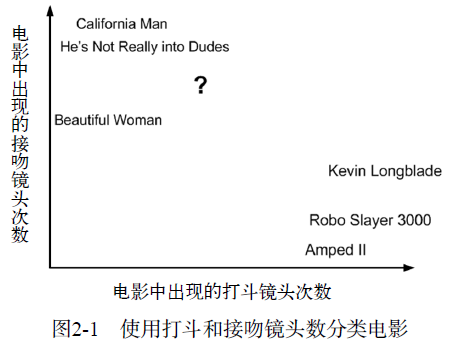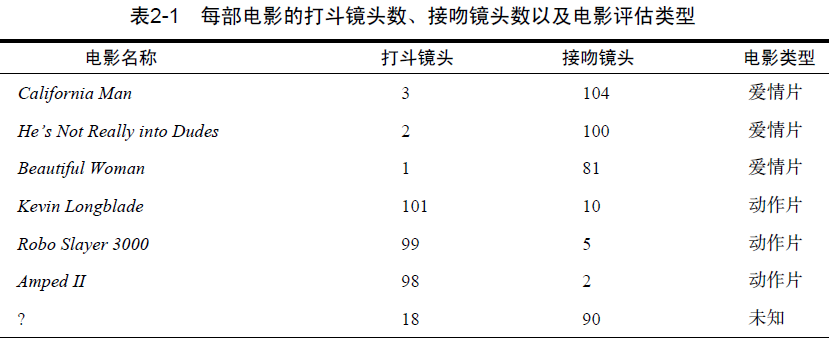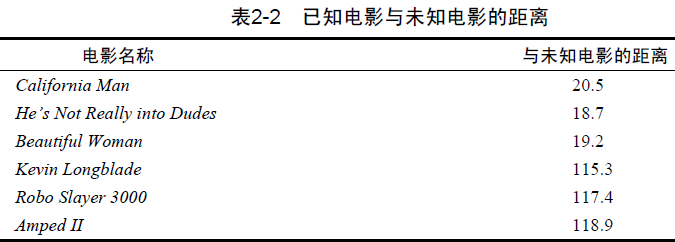k-近邻算法的一般流程
(1) 收集数据：可以使用任何方法。
(2) 准备数据：距离计算所需要的数值，最好是结构化的数据格式。
(3) 分析数据：可以使用任何方法。
(4) 训练算法：此步骤不适用于k-近邻算法。
(5) 测试算法：计算错误率。
(6) 使用算法：首先需要输入样本数据和结构化的输出结果，然后运行k-近邻算法判定输入数据分别属于哪个分类，最后应用对计算出的分类执行后续的处理。

1. 准备：使用Python 导入数据

``````import numpy as np

"""
Parameters:
无
Returns:
group - 数据集
labels - 分类标签
"""
# 函数说明:创建数据集
def createDataSet():
#六组二维特征
group = np.array([[3,104],[2,100],[1,81],[101,10],[99,5],[98,2]])
#六组特征的标签
labels = ['爱情片','爱情片','爱情片','动作片','动作片','动作片']
return group, labels

def showDataSet(dataMat, labelMat):
data_plus = []
for i in range(len(group)):
data_plus.append(dataMat[i])
data_plus_np = np.array(data_plus)# 转换为numpy矩阵
plt.scatter(np.transpose(data_plus_np),np.transpose(data_plus_np))#散点图
plt.show()

if __name__ == '__main__':
#创建数据集
group, labels = createDataSet()
#打印数据集
print(group)
print(labels)
``````
``````>>>
[[  3 104]
[  2 100]
[  1  81]
[101  10]
[ 99   5]
[ 98   2]]
['爱情片', '爱情片', '爱情片', '动作片', '动作片', '动作片']
``````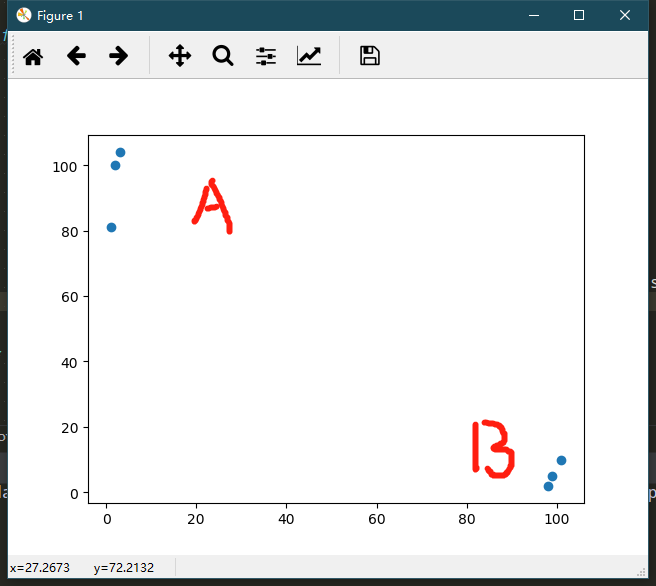1. 实施kNN 算法

(1) 计算已知类别数据集中的点与当前点之间的距离；
(2) 按照距离递增次序排序；
(3) 选取与当前点距离最小的k个点；
(4) 确定前k个点所在类别的出现频率；
(5) 返回前k个点出现频率最高的类别作为当前点的预测分类。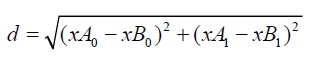``````import numpy as np
import operator

"""
Parameters:
inX - 用于分类的数据(测试集)
dataSet - 用于训练的数据(训练集)
labes - 分类标签
k - kNN算法参数,选择距离最小的k个点
Returns:
sortedClassCount - 分类结果
"""
# 函数说明:kNN算法,分类器
def classify0(inX, dataSet, labels, k):、
#numpy函数shape返回dataSet的行数
dataSetSize = dataSet.shape
#在列向量方向上重复inX共1次(横向)，行向量方向上重复inX共dataSetSize次(纵向)
diffMat = np.tile(inX, (dataSetSize, 1)) - dataSet
#二维特征相减后平方
sqDiffMat = diffMat**2
#sum()所有元素相加，sum(0)列相加，sum(1)行相加
sqDistances = sqDiffMat.sum(axis=1)
#开方，计算出距离
distances = sqDistances**0.5
#返回distances中元素从小到大排序后的索引值
sortedDistIndices = distances.argsort()
#定一个记录类别次数的字典
classCount = {}
for i in range(k):
#取出前k个元素的类别
voteIlabel = labels[sortedDistIndices[i]]
#dict.get(key,default=None),字典的get()方法,返回指定键的值,如果值不在字典中返回默认值。
#计算类别次数
classCount[voteIlabel] = classCount.get(voteIlabel,0) + 1
#python3中用items()替换python2中的iteritems()
#key=operator.itemgetter(1)根据字典的值进行排序
#key=operator.itemgetter(0)根据字典的键进行排序
#reverse降序排序字典
sortedClassCount = sorted(classCount.items(),key=operator.itemgetter(1),reverse=True)
#返回次数最多的类别,即所要分类的类别
return sortedClassCount
``````

``````import numpy as np
import operator

"""
Parameters:
无
Returns:
group - 数据集
labels - 分类标签
"""
# 函数说明:创建数据集
def createDataSet():
#六组二维特征
group = np.array([[3,104],[2,100],[1,81],[101,10],[99,5],[98,2]])
#六组特征的标签
labels = ['爱情片','爱情片','爱情片','动作片','动作片','动作片']
return group, labels

"""
Parameters:
inX - 用于分类的数据(测试集)
dataSet - 用于训练的数据(训练集)
labes - 分类标签
k - kNN算法参数,选择距离最小的k个点
Returns:
sortedClassCount - 分类结果
"""
# 函数说明:kNN算法,分类器
def classify0(inX, dataSet, labels, k):
#numpy函数shape返回dataSet的行数
dataSetSize = dataSet.shape
#在列向量方向上重复inX共1次(横向)，行向量方向上重复inX共dataSetSize次(纵向)
diffMat = np.tile(inX, (dataSetSize, 1)) - dataSet
#二维特征相减后平方
sqDiffMat = diffMat**2
#sum()所有元素相加，sum(0)列相加，sum(1)行相加
sqDistances = sqDiffMat.sum(axis=1)
#开方，计算出距离
distances = sqDistances**0.5
#返回distances中元素从小到大排序后的索引值
sortedDistIndices = distances.argsort()
#定一个记录类别次数的字典
classCount = {}
for i in range(k):
#取出前k个元素的类别
voteIlabel = labels[sortedDistIndices[i]]
#dict.get(key,default=None),字典的get()方法,返回指定键的值,如果值不在字典中返回默认值。
#计算类别次数
classCount[voteIlabel] = classCount.get(voteIlabel,0) + 1
#python3中用items()替换python2中的iteritems()
#key=operator.itemgetter(1)根据字典的值进行排序
#key=operator.itemgetter(0)根据字典的键进行排序
#reverse降序排序字典
sortedClassCount = sorted(classCount.items(),key=operator.itemgetter(1),reverse=True)
#返回次数最多的类别,即所要分类的类别
return sortedClassCount

if __name__ == '__main__':
#创建数据集
group, labels = createDataSet()
#测试集
test = [101,20]
#kNN分类
test_class = classify0(test, group, labels, 3)
#打印分类结果
print(test_class)
``````
``````>>>

``````
1. 如何测试分类器

### 2、使用k-近邻算法改进约会网站的配对效果

(1) 收集数据：提供文本文件。
(2) 准备数据：使用Python解析文本文件。
(3) 分析数据：使用Matplotlib画二维扩散图。
(4) 训练算法：此步骤不适用于k-近邻算法。
(5) 测试算法：使用海伦提供的部分数据作为测试样本。测试样本和非测试样本的区别在于：测试样本是已经完成分类的数据，如果预测分类与实际类别不同，则标记为一个错误。
(6) 使用算法：产生简单的命令行程序，然后海伦可以输入一些特征数据以判断对方是否为自己喜欢的类型。

1. 准备数据：从文本文件中解析数据

• 每年获得的飞行常客里程数
• 玩视频游戏所耗时间百分比
• 每周消费的冰淇淋公升数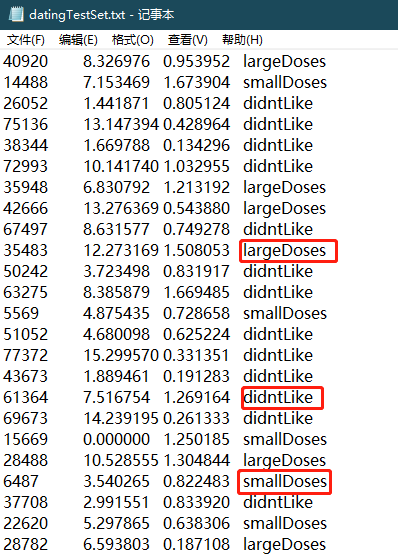``````import numpy as np

"""
Parameters:
filename - 文件名
Returns:
returnMat - 特征矩阵
classLabelVector - 分类Label向量
"""
# 函数说明:打开并解析文件，对数据进行分类：1代表不喜欢,2代表魅力一般,3代表极具魅力
def file2matrix(filename):
#打开文件
fr = open(filename)
#读取文件所有内容
#得到文件行数
numberOfLines = len(arrayOLines)
#返回的NumPy矩阵,解析完成的数据:numberOfLines行,3列
returnMat = np.zeros((numberOfLines,3))
#返回的分类标签向量
classLabelVector = []
#行的索引值
index = 0
for line in arrayOLines:
#s.strip(rm)，当rm空时,默认删除空白符(包括'\n','\r','\t',' ')
line = line.strip()
#使用s.split(str="",num=string,cout(str))将字符串根据'\t'分隔符进行切片。
listFromLine = line.split('\t')
#将数据前三列提取出来,存放到returnMat的NumPy矩阵中,也就是特征矩阵
returnMat[index,:] = listFromLine[0:3]
#根据文本中标记的喜欢的程度进行分类,1代表不喜欢,2代表魅力一般,3代表极具魅力
if listFromLine[-1] == 'didntLike':
classLabelVector.append(1)
elif listFromLine[-1] == 'smallDoses':
classLabelVector.append(2)
elif listFromLine[-1] == 'largeDoses':
classLabelVector.append(3)
index += 1
return returnMat, classLabelVector

if __name__ == '__main__':
#打开的文件名
filename = "datingTestSet.txt"
#打开并处理数据
datingDataMat, datingLabels = file2matrix(filename)
print(datingDataMat)
print(datingLabels)
``````
``````>>>
[[  4.09200000e+04   8.32697600e+00   9.53952000e-01]
[  1.44880000e+04   7.15346900e+00   1.67390400e+00]
[  2.60520000e+04   1.44187100e+00   8.05124000e-01]
...,
[  2.65750000e+04   1.06501020e+01   8.66627000e-01]
[  4.81110000e+04   9.13452800e+00   7.28045000e-01]
[  4.37570000e+04   7.88260100e+00   1.33244600e+00]]
[3, 2, 1, 1, 1, 1, 3, 3, 1, 3, 1, 1, 2, 1, 1, 1, 1, 1, 2, 3, 2, 1, 2, 3, 2, 3, 2, 3, 2, 1, 3, 1, 3, 1, 2, 1, 1, 2, 3, 3, 1, 2, 3, 3, 3, 1, 1, 1, 1, 2, 2, 1, 3, 2, 2, 2, 2, 3, 1, 2, 1, 2, 2, 2, 2, 2, 3, 2, 3, 1, 2, 3, 2, 2, 1, 3, 1, 1, 3, 3, 1, 2, 3, 1, 3, 1, 2, 2, 1, 1, 3, 3, 1, 2, 1, 3, 3, 2, 1, 1, 3, 1, 2, 3, 3, 2, 3, 3, 1, 2, 3, 2, 1, 3, 1, 2, 1, 1, 2, 3, 2, 3, 2, 3, 2, 1, 3, 3, 3, 1, 3, 2, 2, 3, 1, 3, 3, 3, 1, 3, 1, 1, 3, 3, 2, 3, 3, 1, 2, 3, 2, 2, 3, 3, 3, 1, 2, 2, 1, 1, 3, 2, 3, 3, 1, 2, 1, 3, 1, 2, 3, 2, 3, 1, 1, 1, 3, 2, 3, 1, 3, 2, 1, 3, 2, 2, 3, 2, 3, 2, 1, 1, 3, 1, 3, 2, 2, 2, 3, 2, 2, 1, 2, 2, 3, 1, 3, 3, 2, 1, 1, 1, 2, 1, 3, 3, 3, 3, 2, 1, 1, 1, 2, 3, 2, 1, 3, 1, 3, 2, 2, 3, 1, 3, 1, 1, 2, 1, 2, 2, 1, 3, 1, 3, 2, 3, 1, 2, 3, 1, 1, 1, 1, 2, 3, 2, 2, 3, 1, 2, 1, 1, 1, 3, 3, 2, 1, 1, 1, 2, 2, 3, 1, 1, 1, 2, 1, 1, 2, 1, 1, 1, 2, 2, 3, 2, 3, 3, 3, 3, 1, 2, 3, 1, 1, 1, 3, 1, 3, 2, 2, 1, 3, 1, 3, 2, 2, 1, 2, 2, 3, 1, 3, 2, 1, 1, 3, 3, 2, 3, 3, 2, 3, 1, 3, 1, 3, 3, 1, 3, 2, 1, 3, 1, 3, 2, 1, 2, 2, 1, 3, 1, 1, 3, 3, 2, 2, 3, 1, 2, 3, 3, 2, 2, 1, 1, 1, 1, 3, 2, 1, 1, 3, 2, 1, 1, 3, 3, 3, 2, 3, 2, 1, 1, 1, 1, 1, 3, 2, 2, 1, 2, 1, 3, 2, 1, 3, 2, 1, 3, 1, 1, 3, 3, 3, 3, 2, 1, 1, 2, 1, 3, 3, 2, 1, 2, 3, 2, 1, 2, 2, 2, 1, 1, 3, 1, 1, 2, 3, 1, 1, 2, 3, 1, 3, 1, 1, 2, 2, 1, 2, 2, 2, 3, 1, 1, 1, 3, 1, 3, 1, 3, 3, 1, 1, 1, 3, 2, 3, 3, 2, 2, 1, 1, 1, 2, 1, 2, 2, 3, 3, 3, 1, 1, 3, 3, 2, 3, 3, 2, 3, 3, 3, 2, 3, 3, 1, 2, 3, 2, 1, 1, 1, 1, 3, 3, 3, 3, 2, 1, 1, 1, 1, 3, 1, 1, 2, 1, 1, 2, 3, 2, 1, 2, 2, 2, 3, 2, 1, 3, 2, 3, 2, 3, 2, 1, 1, 2, 3, 1, 3, 3, 3, 1, 2, 1, 2, 2, 1, 2, 2, 2, 2, 2, 3, 2, 1, 3, 3, 2, 2, 2, 3, 1, 2, 1, 1, 3, 2, 3, 2, 3, 2, 3, 3, 2, 2, 1, 3, 1, 2, 1, 3, 1, 1, 1, 3, 1, 1, 3, 3, 2, 2, 1, 3, 1, 1, 3, 2, 3, 1, 1, 3, 1, 3, 3, 1, 2, 3, 1, 3, 1, 1, 2, 1, 3, 1, 1, 1, 1, 2, 1, 3, 1, 2, 1, 3, 1, 3, 1, 1, 2, 2, 2, 3, 2, 2, 1, 2, 3, 3, 2, 3, 3, 3, 2, 3, 3, 1, 3, 2, 3, 2, 1, 2, 1, 1, 1, 2, 3, 2, 2, 1, 2, 2, 1, 3, 1, 3, 3, 3, 2, 2, 3, 3, 1, 2, 2, 2, 3, 1, 2, 1, 3, 1, 2, 3, 1, 1, 1, 2, 2, 3, 1, 3, 1, 1, 3, 1, 2, 3, 1, 2, 3, 1, 2, 3, 2, 2, 2, 3, 1, 3, 1, 2, 3, 2, 2, 3, 1, 2, 3, 2, 3, 1, 2, 2, 3, 1, 1, 1, 2, 2, 1, 1, 2, 1, 2, 1, 2, 3, 2, 1, 3, 3, 3, 1, 1, 3, 1, 2, 3, 3, 2, 2, 2, 1, 2, 3, 2, 2, 3, 2, 2, 2, 3, 3, 2, 1, 3, 2, 1, 3, 3, 1, 2, 3, 2, 1, 3, 3, 3, 1, 2, 2, 2, 3, 2, 3, 3, 1, 2, 1, 1, 2, 1, 3, 1, 2, 2, 1, 3, 2, 1, 3, 3, 2, 2, 2, 1, 2, 2, 1, 3, 1, 3, 1, 3, 3, 1, 1, 2, 3, 2, 2, 3, 1, 1, 1, 1, 3, 2, 2, 1, 3, 1, 2, 3, 1, 3, 1, 3, 1, 1, 3, 2, 3, 1, 1, 3, 3, 3, 3, 1, 3, 2, 2, 1, 1, 3, 3, 2, 2, 2, 1, 2, 1, 2, 1, 3, 2, 1, 2, 2, 3, 1, 2, 2, 2, 3, 2, 1, 2, 1, 2, 3, 3, 2, 3, 1, 1, 3, 3, 1, 2, 2, 2, 2, 2, 2, 1, 3, 3, 3, 3, 3, 1, 1, 3, 2, 1, 2, 1, 2, 2, 3, 2, 2, 2, 3, 1, 2, 1, 2, 2, 1, 1, 2, 3, 3, 1, 1, 1, 1, 3, 3, 3, 3, 3, 3, 1, 3, 3, 2, 3, 2, 3, 3, 2, 2, 1, 1, 1, 3, 3, 1, 1, 1, 3, 3, 2, 1, 2, 1, 1, 2, 2, 1, 1, 1, 3, 1, 1, 2, 3, 2, 2, 1, 3, 1, 2, 3, 1, 2, 2, 2, 2, 3, 2, 3, 3, 1, 2, 1, 2, 3, 1, 3, 2, 2, 2, 2, 2, 2, 2, 2, 2, 2, 2, 2, 3, 2, 2, 2, 2, 2, 1, 3, 3, 3]
``````

1. 分析数据：使用Matplotlib 创建散点图

``````from matplotlib.font_manager import FontProperties
import matplotlib.lines as mlines
import matplotlib.pyplot as plt
import numpy as np

"""
Parameters:
filename - 文件名
Returns:
returnMat - 特征矩阵
classLabelVector - 分类Label向量
"""
# 函数说明:打开并解析文件，对数据进行分类：1代表不喜欢,2代表魅力一般,3代表极具魅力
def file2matrix(filename):
#打开文件
fr = open(filename)
#读取文件所有内容
#得到文件行数
numberOfLines = len(arrayOLines)
#返回的NumPy矩阵,解析完成的数据:numberOfLines行,3列
returnMat = np.zeros((numberOfLines,3))
#返回的分类标签向量
classLabelVector = []
#行的索引值
index = 0
for line in arrayOLines:
#s.strip(rm)，当rm空时,默认删除空白符(包括'\n','\r','\t',' ')
line = line.strip()
#使用s.split(str="",num=string,cout(str))将字符串根据'\t'分隔符进行切片。
listFromLine = line.split('\t')
#将数据前三列提取出来,存放到returnMat的NumPy矩阵中,也就是特征矩阵
returnMat[index,:] = listFromLine[0:3]
#根据文本中标记的喜欢的程度进行分类,1代表不喜欢,2代表魅力一般,3代表极具魅力
if listFromLine[-1] == 'didntLike':
classLabelVector.append(1)
elif listFromLine[-1] == 'smallDoses':
classLabelVector.append(2)
elif listFromLine[-1] == 'largeDoses':
classLabelVector.append(3)
index += 1
return returnMat, classLabelVector

"""
Parameters:
datingDataMat - 特征矩阵
datingLabels - 分类Label
Returns:
无
"""
# 函数说明:可视化数据
def showdatas(datingDataMat, datingLabels):
#设置汉字格式
font = FontProperties(fname=r"c:\windows\fonts\simsun.ttc", size=14)
#将fig画布分隔成1行1列,不共享x轴和y轴,fig画布的大小为(13,8)
#当nrow=2,nclos=2时,代表fig画布被分为四个区域,axs表示第一行第一个区域
fig, axs = plt.subplots(nrows=2, ncols=2,sharex=False, sharey=False, figsize=(13,8))

numberOfLabels = len(datingLabels)
LabelsColors = []
for i in datingLabels:
if i == 1:
LabelsColors.append('black')
if i == 2:
LabelsColors.append('orange')
if i == 3:
LabelsColors.append('red')
#画出散点图,以datingDataMat矩阵的第一(飞行常客例程)、第二列(玩游戏)数据画散点数据,散点大小为15,透明度为0.5
axs.scatter(x=datingDataMat[:,0], y=datingDataMat[:,1], color=LabelsColors,s=15, alpha=.5)
#设置标题,x轴label,y轴label
axs0_title_text = axs.set_title(u'每年获得的飞行常客里程数与玩视频游戏所消耗时间占比',FontProperties=font)
axs0_xlabel_text = axs.set_xlabel(u'每年获得的飞行常客里程数',FontProperties=font)
axs0_ylabel_text = axs.set_ylabel(u'玩视频游戏所消耗时间占',FontProperties=font)
plt.setp(axs0_title_text, size=9, weight='bold', color='red')
plt.setp(axs0_xlabel_text, size=7, weight='bold', color='black')
plt.setp(axs0_ylabel_text, size=7, weight='bold', color='black')

#画出散点图,以datingDataMat矩阵的第一(飞行常客例程)、第三列(冰激凌)数据画散点数据,散点大小为15,透明度为0.5
axs.scatter(x=datingDataMat[:,0], y=datingDataMat[:,2], color=LabelsColors,s=15, alpha=.5)
#设置标题,x轴label,y轴label
axs1_title_text = axs.set_title(u'每年获得的飞行常客里程数与每周消费的冰激淋公升数',FontProperties=font)
axs1_xlabel_text = axs.set_xlabel(u'每年获得的飞行常客里程数',FontProperties=font)
axs1_ylabel_text = axs.set_ylabel(u'每周消费的冰激淋公升数',FontProperties=font)
plt.setp(axs1_title_text, size=9, weight='bold', color='red')
plt.setp(axs1_xlabel_text, size=7, weight='bold', color='black')
plt.setp(axs1_ylabel_text, size=7, weight='bold', color='black')

#画出散点图,以datingDataMat矩阵的第二(玩游戏)、第三列(冰激凌)数据画散点数据,散点大小为15,透明度为0.5
axs.scatter(x=datingDataMat[:,1], y=datingDataMat[:,2], color=LabelsColors,s=15, alpha=.5)
#设置标题,x轴label,y轴label
axs2_title_text = axs.set_title(u'玩视频游戏所消耗时间占比与每周消费的冰激淋公升数',FontProperties=font)
axs2_xlabel_text = axs.set_xlabel(u'玩视频游戏所消耗时间占比',FontProperties=font)
axs2_ylabel_text = axs.set_ylabel(u'每周消费的冰激淋公升数',FontProperties=font)
plt.setp(axs2_title_text, size=9, weight='bold', color='red')
plt.setp(axs2_xlabel_text, size=7, weight='bold', color='black')
plt.setp(axs2_ylabel_text, size=7, weight='bold', color='black')
#设置图例
didntLike = mlines.Line2D([], [], color='black', marker='.',
markersize=6, label='didntLike')
smallDoses = mlines.Line2D([], [], color='orange', marker='.',
markersize=6, label='smallDoses')
largeDoses = mlines.Line2D([], [], color='red', marker='.',
markersize=6, label='largeDoses')
#添加图例
axs.legend(handles=[didntLike,smallDoses,largeDoses])
axs.legend(handles=[didntLike,smallDoses,largeDoses])
axs.legend(handles=[didntLike,smallDoses,largeDoses])
#显示图片
plt.show()

if __name__ == '__main__':
#打开的文件名
filename = "datingTestSet.txt"
#打开并处理数据
datingDataMat, datingLabels = file2matrix(filename)
showdatas(datingDataMat, datingLabels)
``````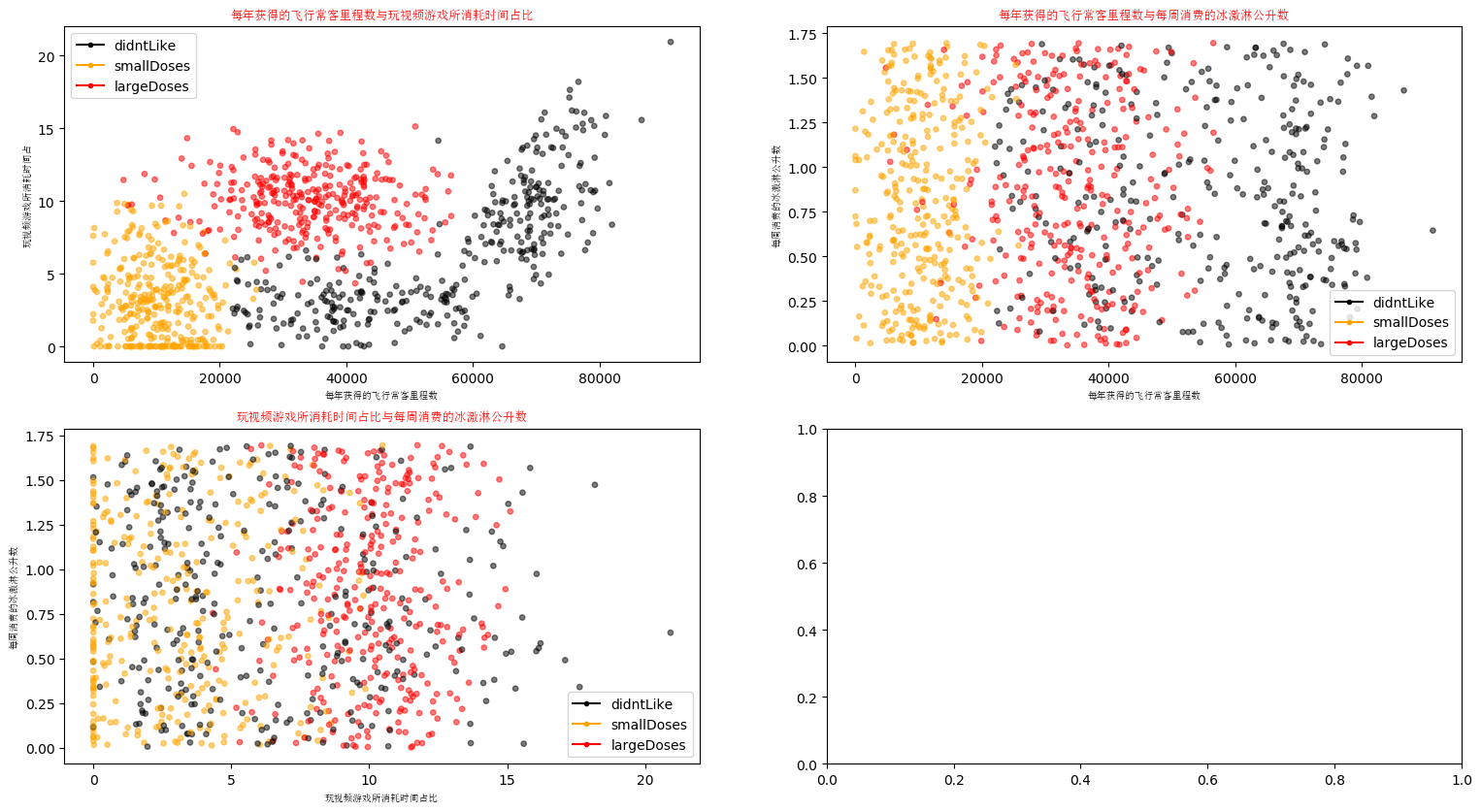1. 准备数据：归一化数值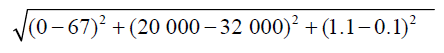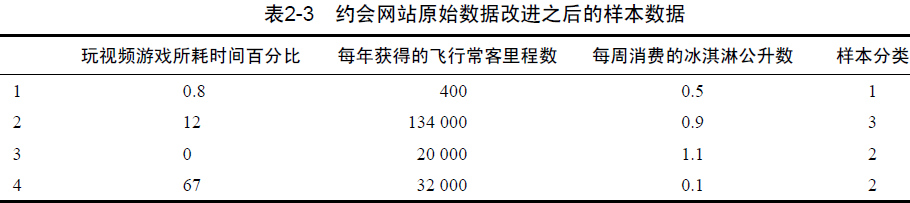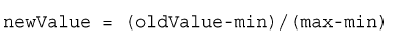``````import numpy as np

"""
Parameters:
filename - 文件名
Returns:
returnMat - 特征矩阵
classLabelVector - 分类Label向量
"""
# 函数说明:打开并解析文件，对数据进行分类：1代表不喜欢,2代表魅力一般,3代表极具魅力
def file2matrix(filename):
#打开文件
fr = open(filename)
#读取文件所有内容
#得到文件行数
numberOfLines = len(arrayOLines)
#返回的NumPy矩阵,解析完成的数据:numberOfLines行,3列
returnMat = np.zeros((numberOfLines,3))
#返回的分类标签向量
classLabelVector = []
#行的索引值
index = 0
for line in arrayOLines:
#s.strip(rm)，当rm空时,默认删除空白符(包括'\n','\r','\t',' ')
line = line.strip()
#使用s.split(str="",num=string,cout(str))将字符串根据'\t'分隔符进行切片。
listFromLine = line.split('\t')
#将数据前三列提取出来,存放到returnMat的NumPy矩阵中,也就是特征矩阵
returnMat[index,:] = listFromLine[0:3]
#根据文本中标记的喜欢的程度进行分类,1代表不喜欢,2代表魅力一般,3代表极具魅力
if listFromLine[-1] == 'didntLike':
classLabelVector.append(1)
elif listFromLine[-1] == 'smallDoses':
classLabelVector.append(2)
elif listFromLine[-1] == 'largeDoses':
classLabelVector.append(3)
index += 1
return returnMat, classLabelVector

"""
Parameters:
dataSet - 特征矩阵
Returns:
normDataSet - 归一化后的特征矩阵
ranges - 数据范围
minVals - 数据最小值
"""
# 函数说明:对数据进行归一化
def autoNorm(dataSet):
#获得数据的最小值
minVals = dataSet.min(0)
maxVals = dataSet.max(0)
#最大值和最小值的范围
ranges = maxVals - minVals
#shape(dataSet)返回dataSet的矩阵行列数
normDataSet = np.zeros(np.shape(dataSet))
#返回dataSet的行数
m = dataSet.shape
#原始值减去最小值
normDataSet = dataSet - np.tile(minVals, (m, 1))
#除以最大和最小值的差,得到归一化数据
normDataSet = normDataSet / np.tile(ranges, (m, 1))
#返回归一化数据结果,数据范围,最小值
return normDataSet, ranges, minVals

if __name__ == '__main__':
#打开的文件名
filename = "datingTestSet.txt"
#打开并处理数据
datingDataMat, datingLabels = file2matrix(filename)
normDataSet, ranges, minVals = autoNorm(datingDataMat)
print(normDataSet)
print(ranges)
print(minVals)
``````
``````>>>
[[ 0.44832535  0.39805139  0.56233353]
[ 0.15873259  0.34195467  0.98724416]
[ 0.28542943  0.06892523  0.47449629]
...,
[ 0.29115949  0.50910294  0.51079493]
[ 0.52711097  0.43665451  0.4290048 ]
[ 0.47940793  0.3768091   0.78571804]]
[  9.12730000e+04   2.09193490e+01   1.69436100e+00]
[ 0.        0.        0.001156]
``````

1. 测试算法：作为完整程序验证分类器

``````import numpy as np
import operator

"""
Parameters:
inX - 用于分类的数据(测试集)
dataSet - 用于训练的数据(训练集)
labes - 分类标签
k - kNN算法参数,选择距离最小的k个点
Returns:
sortedClassCount - 分类结果
"""
# 函数说明:kNN算法,分类器
def classify0(inX, dataSet, labels, k):
#numpy函数shape返回dataSet的行数
dataSetSize = dataSet.shape
#在列向量方向上重复inX共1次(横向),行向量方向上重复inX共dataSetSize次(纵向)
diffMat = np.tile(inX, (dataSetSize, 1)) - dataSet
#二维特征相减后平方
sqDiffMat = diffMat**2
#sum()所有元素相加,sum(0)列相加,sum(1)行相加
sqDistances = sqDiffMat.sum(axis=1)
#开方,计算出距离
distances = sqDistances**0.5
#返回distances中元素从小到大排序后的索引值
sortedDistIndices = distances.argsort()
#定一个记录类别次数的字典
classCount = {}
for i in range(k):
#取出前k个元素的类别
voteIlabel = labels[sortedDistIndices[i]]
#dict.get(key,default=None),字典的get()方法,返回指定键的值,如果值不在字典中返回默认值。
#计算类别次数
classCount[voteIlabel] = classCount.get(voteIlabel,0) + 1
#python3中用items()替换python2中的iteritems()
#key=operator.itemgetter(1)根据字典的值进行排序
#key=operator.itemgetter(0)根据字典的键进行排序
#reverse降序排序字典
sortedClassCount = sorted(classCount.items(),key=operator.itemgetter(1),reverse=True)
#返回次数最多的类别,即所要分类的类别
return sortedClassCount

"""
Parameters:
filename - 文件名
Returns:
returnMat - 特征矩阵
classLabelVector - 分类Label向量
"""
# 函数说明:打开并解析文件，对数据进行分类：1代表不喜欢,2代表魅力一般,3代表极具魅力
def file2matrix(filename):
#打开文件
fr = open(filename)
#读取文件所有内容
#得到文件行数
numberOfLines = len(arrayOLines)
#返回的NumPy矩阵,解析完成的数据:numberOfLines行,3列
returnMat = np.zeros((numberOfLines,3))
#返回的分类标签向量
classLabelVector = []
#行的索引值
index = 0
for line in arrayOLines:
#s.strip(rm)，当rm空时,默认删除空白符(包括'\n','\r','\t',' ')
line = line.strip()
#使用s.split(str="",num=string,cout(str))将字符串根据'\t'分隔符进行切片。
listFromLine = line.split('\t')
#将数据前三列提取出来,存放到returnMat的NumPy矩阵中,也就是特征矩阵
returnMat[index,:] = listFromLine[0:3]
#根据文本中标记的喜欢的程度进行分类,1代表不喜欢,2代表魅力一般,3代表极具魅力
if listFromLine[-1] == 'didntLike':
classLabelVector.append(1)
elif listFromLine[-1] == 'smallDoses':
classLabelVector.append(2)
elif listFromLine[-1] == 'largeDoses':
classLabelVector.append(3)
index += 1
return returnMat, classLabelVector

"""
Parameters:
dataSet - 特征矩阵
Returns:
normDataSet - 归一化后的特征矩阵
ranges - 数据范围
minVals - 数据最小值
"""
# 函数说明:对数据进行归一化
def autoNorm(dataSet):
#获得数据的最小值
minVals = dataSet.min(0)
maxVals = dataSet.max(0)
#最大值和最小值的范围
ranges = maxVals - minVals
#shape(dataSet)返回dataSet的矩阵行列数
normDataSet = np.zeros(np.shape(dataSet))
#返回dataSet的行数
m = dataSet.shape
#原始值减去最小值
normDataSet = dataSet - np.tile(minVals, (m, 1))
#除以最大和最小值的差,得到归一化数据
normDataSet = normDataSet / np.tile(ranges, (m, 1))
#返回归一化数据结果,数据范围,最小值
return normDataSet, ranges, minVals

"""
Parameters:
无
Returns:
normDataSet - 归一化后的特征矩阵
ranges - 数据范围
minVals - 数据最小值
"""
# 函数说明:分类器测试函数
def datingClassTest():
#打开的文件名
filename = "datingTestSet.txt"
#将返回的特征矩阵和分类向量分别存储到datingDataMat和datingLabels中
datingDataMat, datingLabels = file2matrix(filename)
#取所有数据的百分之十
hoRatio = 0.10
#数据归一化,返回归一化后的矩阵,数据范围,数据最小值
normMat, ranges, minVals = autoNorm(datingDataMat)
#获得normMat的行数
m = normMat.shape
#百分之十的测试数据的个数
numTestVecs = int(m * hoRatio)
#分类错误计数
errorCount = 0.0

for i in range(numTestVecs):
#前numTestVecs个数据作为测试集,后m-numTestVecs个数据作为训练集
classifierResult = classify0(normMat[i,:], normMat[numTestVecs:m,:],
datingLabels[numTestVecs:m], 4)
print("分类结果:%d\t真实类别:%d" % (classifierResult, datingLabels[i]))
if classifierResult != datingLabels[i]:
errorCount += 1.0
print("错误率:%f%%" %(errorCount/float(numTestVecs)*100))

if __name__ == '__main__':
datingClassTest()
``````
``````>>>
......
......

``````

1. 使用算法：构建完整可用系统

``````import numpy as np
import operator

"""
Parameters:
inX - 用于分类的数据(测试集)
dataSet - 用于训练的数据(训练集)
labes - 分类标签
k - kNN算法参数,选择距离最小的k个点
Returns:
sortedClassCount - 分类结果
"""
# 函数说明:kNN算法,分类器
def classify0(inX, dataSet, labels, k):
#numpy函数shape返回dataSet的行数
dataSetSize = dataSet.shape
#在列向量方向上重复inX共1次(横向),行向量方向上重复inX共dataSetSize次(纵向)
diffMat = np.tile(inX, (dataSetSize, 1)) - dataSet
#二维特征相减后平方
sqDiffMat = diffMat**2
#sum()所有元素相加,sum(0)列相加,sum(1)行相加
sqDistances = sqDiffMat.sum(axis=1)
#开方,计算出距离
distances = sqDistances**0.5
#返回distances中元素从小到大排序后的索引值
sortedDistIndices = distances.argsort()
#定一个记录类别次数的字典
classCount = {}
for i in range(k):
#取出前k个元素的类别
voteIlabel = labels[sortedDistIndices[i]]
#dict.get(key,default=None),字典的get()方法,返回指定键的值,如果值不在字典中返回默认值。
#计算类别次数
classCount[voteIlabel] = classCount.get(voteIlabel,0) + 1
#python3中用items()替换python2中的iteritems()
#key=operator.itemgetter(1)根据字典的值进行排序
#key=operator.itemgetter(0)根据字典的键进行排序
#reverse降序排序字典
sortedClassCount = sorted(classCount.items(),key=operator.itemgetter(1),reverse=True)
#返回次数最多的类别,即所要分类的类别
return sortedClassCount

"""
Parameters:
filename - 文件名
Returns:
returnMat - 特征矩阵
classLabelVector - 分类Label向量
"""
# 函数说明:打开并解析文件，对数据进行分类：1代表不喜欢,2代表魅力一般,3代表极具魅力
def file2matrix(filename):
#打开文件
fr = open(filename)
#读取文件所有内容
#得到文件行数
numberOfLines = len(arrayOLines)
#返回的NumPy矩阵,解析完成的数据:numberOfLines行,3列
returnMat = np.zeros((numberOfLines,3))
#返回的分类标签向量
classLabelVector = []
#行的索引值
index = 0
for line in arrayOLines:
#s.strip(rm)，当rm空时,默认删除空白符(包括'\n','\r','\t',' ')
line = line.strip()
#使用s.split(str="",num=string,cout(str))将字符串根据'\t'分隔符进行切片。
listFromLine = line.split('\t')
#将数据前三列提取出来,存放到returnMat的NumPy矩阵中,也就是特征矩阵
returnMat[index,:] = listFromLine[0:3]
#根据文本中标记的喜欢的程度进行分类,1代表不喜欢,2代表魅力一般,3代表极具魅力
if listFromLine[-1] == 'didntLike':
classLabelVector.append(1)
elif listFromLine[-1] == 'smallDoses':
classLabelVector.append(2)
elif listFromLine[-1] == 'largeDoses':
classLabelVector.append(3)
index += 1
return returnMat, classLabelVector

"""
Parameters:
dataSet - 特征矩阵
Returns:
normDataSet - 归一化后的特征矩阵
ranges - 数据范围
minVals - 数据最小值
"""
# 函数说明:对数据进行归一化
def autoNorm(dataSet):
#获得数据的最小值
minVals = dataSet.min(0)
maxVals = dataSet.max(0)
#最大值和最小值的范围
ranges = maxVals - minVals
#shape(dataSet)返回dataSet的矩阵行列数
normDataSet = np.zeros(np.shape(dataSet))
#返回dataSet的行数
m = dataSet.shape
#原始值减去最小值
normDataSet = dataSet - np.tile(minVals, (m, 1))
#除以最大和最小值的差,得到归一化数据
normDataSet = normDataSet / np.tile(ranges, (m, 1))
#返回归一化数据结果,数据范围,最小值
return normDataSet, ranges, minVals

# 函数说明:通过输入一个人的三维特征,进行分类输出
def classifyPerson():
#输出结果
resultList = ['讨厌','有些喜欢','非常喜欢']
#三维特征用户输入
precentTats = float(input("玩视频游戏所耗时间百分比:"))
ffMiles = float(input("每年获得的飞行常客里程数:"))
iceCream = float(input("每周消费的冰激淋公升数:"))
#打开的文件名
filename = "datingTestSet.txt"
#打开并处理数据
datingDataMat, datingLabels = file2matrix(filename)
#训练集归一化
normMat, ranges, minVals = autoNorm(datingDataMat)
#生成NumPy数组,测试集
inArr = np.array([precentTats, ffMiles, iceCream])
#测试集归一化
norminArr = (inArr - minVals) / ranges
#返回分类结果
classifierResult = classify0(norminArr, normMat, datingLabels, 3)
#打印结果
print("你可能%s这个人" % (resultList[classifierResult-1]))

if __name__ == '__main__':
classifyPerson()
``````

``````>>>

``````

### 3、Sklearn构建k-近邻分类器用于手写数字识别

(1) 收集数据：提供文本文件。
(2) 准备数据：编写函数classify0()，将图像格式转换为分类器使用的list格式。
(3) 分析数据：在Python命令提示符中检查数据，确保它符合要求。
(4) 训练算法：此步骤不适用于k-近邻算法。
(5) 测试算法：编写函数使用提供的部分数据集作为测试样本，测试样本与非测试样本的区别在于测试样本是已经完成分类的数据，如果预测分类与实际类别不同，则标记为一个错误。
(6) 使用算法：本例没有完成此步骤，若你感兴趣可以构建完整的应用程序，从图像中提取数字，并完成数字识别，美国的邮件分拣系统就是一个实际运行的类似系统。

1. 准备数据：将图像转换为测试向量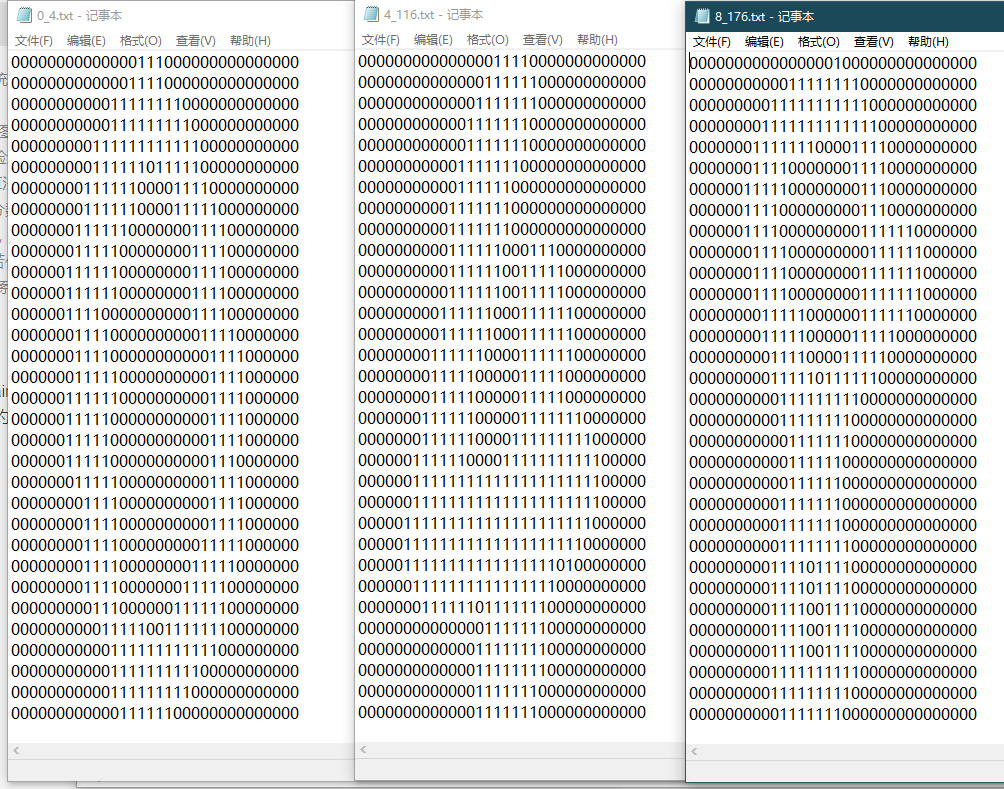1. 测试算法：使用k-近邻算法识别手写数字

``````import numpy as np
import operator
from os import listdir
from sklearn.neighbors import KNeighborsClassifier as kNN

"""
Parameters:
filename - 文件名
Returns:
returnVect - 返回的二进制图像的1x1024向量
"""
# 函数说明:将32x32的二进制图像转换为1x1024向量。
def img2vector(filename):
#创建1x1024零向量
returnVect = np.zeros((1, 1024))
#打开文件
fr = open(filename)
#按行读取
for i in range(32):
#读一行数据
#每一行的前32个元素依次添加到returnVect中
for j in range(32):
returnVect[0, 32*i+j] = int(lineStr[j])
#返回转换后的1x1024向量
return returnVect

# 函数说明:手写数字分类测试
def handwritingClassTest():
#测试集的Labels
hwLabels = []
#返回trainingDigits目录下的文件名
trainingFileList = listdir('trainingDigits')
#返回文件夹下文件的个数
m = len(trainingFileList)
#初始化训练的Mat矩阵,测试集
trainingMat = np.zeros((m, 1024))
#从文件名中解析出训练集的类别
for i in range(m):
#获得文件的名字
fileNameStr = trainingFileList[i]
#获得分类的数字
classNumber = int(fileNameStr.split('_'))
#将获得的类别添加到hwLabels中
hwLabels.append(classNumber)
#将每一个文件的1x1024数据存储到trainingMat矩阵中
trainingMat[i,:] = img2vector('trainingDigits/%s' % (fileNameStr))
#构建kNN分类器
neigh = kNN(n_neighbors = 3, algorithm = 'auto')
#拟合模型, trainingMat为测试矩阵,hwLabels为对应的标签
neigh.fit(trainingMat, hwLabels)
#返回testDigits目录下的文件列表
testFileList = listdir('testDigits')
#错误检测计数
errorCount = 0.0
#测试数据的数量
mTest = len(testFileList)
#从文件中解析出测试集的类别并进行分类测试
for i in range(mTest):
#获得文件的名字
fileNameStr = testFileList[i]
#获得分类的数字
classNumber = int(fileNameStr.split('_'))
#获得测试集的1x1024向量,用于训练
vectorUnderTest = img2vector('testDigits/%s' % (fileNameStr))
#获得预测结果
# classifierResult = classify0(vectorUnderTest, trainingMat, hwLabels, 3)
classifierResult = neigh.predict(vectorUnderTest)
print("分类返回结果为%d\t真实结果为%d" % (classifierResult, classNumber))
if(classifierResult != classNumber):
errorCount += 1.0
print("总共错了%d个数据\n错误率为%f%%" % (errorCount, errorCount/mTest * 100))

if __name__ == '__main__':
handwritingClassTest()
``````
``````>>>

``````

### 4、sklearn.neighbors.KNeighborsClassifier

sklearn.neighbors.KNeighborsClassifier是一个很好的模型，k-近邻算法就是通过它实现的，详细的看这个博客——sklearn.neighbors.KNeighborsClassifier()函数解析

### 5、总结

k-近邻算法是分类数据最简单最有效的算法，通过两个例子（约会网站和手写数字识别）讲述了如何使用k-近邻算法构造分类器。。k-近邻算法是基于实例的学习，使用算法时我们必须有接近实际数据的训练样本数据。k-近邻算法必须保存全部数据集，如果训练数据集的很大，必须使用大量的存储空间。此外，由于必须对数据集中的每个数据计算距离值，实际使用时可能非常耗时。

k-近邻算法的另一个缺陷是它无法给出任何数据的基础结构信息，因此也无法知晓平均实例样本和典型实例样本具有什么特征。下一章我们将使用概率测量方法处理分类问题，该算法可以解决这个问题。

### 参考文章

k算法 机器学习实战 相关内容

2019-11-10 18:13:48 qq_16829085 阅读数 19
• ###### python数据分析与机器学习实战【2019新版】

购买课程后，请扫码进入学习群，获取唐宇迪老师答疑 Python数据分析与机器学习实战教程，该课程精心挑选真实的数据集为案例，通过python数据科学库numpy,pandas,matplot结合机器学习库scikit-learn完成一些列的机器学习案例。课程以实战为基础，所有课时都结合代码演示如何使用这些python库来完成一个真实的数据案例。算法与项目相结合，选择经典kaggle项目，从数据预处理开始一步步代码实战带大家入门机器学习。学完该课程即可： 1.掌握Python数据科学工具包，包括矩阵数据处理与可视化展示。 2.掌握机器学习算法原理推导，从数学上理解算法是怎么来的以及其中涉及的细节。 3.掌握每一个算法所涉及的参数，详解其中每一步对结果的影响。 4.熟练使用Python进行建模实战，基于真实数据集展开分析，一步步完成整个建模实战任务。

93869 人正在学习 去看看 唐宇迪

## 参考资料:

``````'''
@version: 0.0.1
@Author: Huang
@dev: python3 vscode
@Date: 2019-11-10 11:39:30
@LastEditTime: 2019-11-10 17:57:13
@FilePath: \\机器学习实战\\10-K均值聚类算法\\kMeans.py
@Descripttion: 聚类是一种无监督的学习，它将相似的对象归到同一个簇中
'''

import numpy as np
import matplotlib.pyplot as plt

"""
[summary]:加载数据

Arguments:
filename  -- 文件名

Returns:
[List] -- 数据集
"""
dataMat = []
with open(filename) as fr:
curline = line.strip().split()
fltline = list(map(float, curline))
dataMat.append(fltline)
return dataMat

def distEclud(vecA, vecB):
"""
[summary]:计算两个向量的欧氏距离

Arguments:
vecA  -- A坐标
vecB  -- B坐标

Returns:
两点之间的欧氏距离
"""
# not sum(mat) but mat.sum()
return np.sqrt(np.power(vecA - vecB, 2).sum())

def randCent(dataSet, k):
"""
[summary]:为数据集构建k个随机质心的集合

Arguments:
dataSet {[mat]} -- 数据集
k {[int} -- 聚类数

Returns:
[mat] -- k个中心点组成的矩阵
"""
n = np.shape(dataSet)  # 获取列数
centroids = np.mat(np.zeros((k, n)))
# 遍历所有列
for j in range(n):
minj = min(dataSet[:, j])
rangej = float(max(dataSet[:, j]) - minj)
# 最小值+区间×随机系数,确保生成的中心点在数据集边界之内
centroids[:, j] = minj + rangej * np.random.rand(k, 1)
return centroids

def my_KMeans(dataSet, k, distMeas=distEclud, createCent=randCent):
"""
[summary]:
创建k个点作为起始质心（经常是随机选择）
当任意一个点的簇分配结果发生改变时
对数据集中的每个数据点
对每个质心
计算质心与数据点之间的距离
将数据点分配到距其最近的簇
对每一个簇，计算簇中所有点的均值并将均值作为质心

Arguments:
dataSet {[mat]} -- 数据集
k {[int]} -- 聚类数

Keyword Arguments:
distMeas  -- 距离算法 (default: {distEclud})
createCent -- 创建初始质心 (default: {randCent})

Returns:
centroids -- (k,n) 类质心
clusterAssment -- (m,2) 点分配
"""
m = np.shape(dataSet)  # 行数
# 簇分配结果：第一列记录索引值;第二列存储误差,当前点到簇质心的距离
clusterAssment = np.mat(np.zeros((m, 2)))
# 随机生成中心点完成初始化
centroids = randCent(dataSet, k)  # (k,n)
clusterchanged = True
while clusterchanged:
# 假定所有点分配都不发生改变，标记为False
clusterchanged = False
for i in range(m):
cluster_i = clusterAssment[i, 0]  # 取出簇索引值
dismax = np.inf
for j in range(k):
curdis = distEclud(centroids[j, :], dataSet[i, :])
if curdis < dismax:
dismax = curdis
# 更新簇分配结果
clusterAssment[i, :] = j, dismax
if cluster_i != clusterAssment[i, 0]:
clusterchanged = True
print(centroids)
for cent in range(k):
ptsInClust = dataSet[np.nonzero(clusterAssment[:, 0].A == cent)]
# 沿矩阵的列方向进行均值计算
centroids[cent, :] = np.mean(ptsInClust, axis=0)
# 返回所有的类质心与点分配结果
return centroids, clusterAssment

def plt_my_KMeans():
# 对数据进行聚类
centroidsOfData, clusterAssmentOfData = my_KMeans(data, 4)
# 数据集的数量
m = np.shape(data)
# 画出数据的散点图
plt.scatter(data[:, 0].A.reshape(m),
data[:, 1].A.reshape(m),
c=clusterAssmentOfData.A[:, 0].reshape(m))
# 用红色的三角形符号画出聚类中心
plt.scatter(centroidsOfData.A[:, 0],
centroidsOfData.A[:, 1],
c='red',
marker='^')
# 显示图片
plt.show()

def biKmeans(dataSet, k, distMeas=distEclud):
"""
[summary]:二分K-均值算法
将所有点看成一个簇
当簇数目小于k时
对于每一个簇
计算总误差
在给定的簇上面进行K-均值聚类(k=2)
计算将该簇一分为二之后的总误差
选择使得误差最小的那个簇进行划分操作

Arguments:
dataSet {[mat]} -- 数据集
k {[int]} -- 聚类数

Keyword Arguments:
distMeas  -- 距离算法 (default: {distEclud})

Returns:
centroids -- (k,n) 类质心
clusterAssment -- (m,2) 点分配
"""
m, n = np.shape(dataSet)
clusterAssment = np.mat(np.zeros((m, 2)))
# 创建一个初始簇
centroid0 = np.mean(dataSet, axis=0).tolist()
cenList = [centroid0]  # 保留质心
# 计算误差
for j in range(m):
clusterAssment[j, 1] = distMeas(np.mat(centroid0), dataSet[j, :])**2
# 对簇进行划分
while len(cenList) < k:
lowestSSE = np.inf
# 尝试划分每一簇
for i in range(len(cenList)):
#找出正在计算的簇
ptscurrCluster = dataSet[np.nonzero(
clusterAssment[:, 0].A == i), :]
# 对给定簇进行K-均值聚类
centroidMat, splitClustAss = my_KMeans(ptscurrCluster, 2, distMeas)
# 计算划分后的SSE(误差平方和)
ssesplit = np.sum(splitClustAss[:, 1])
# 计算剩余数据集的SSE(误差平方和)
ssenotsplit = np.sum(
clusterAssment[np.nonzero(clusterAssment[:, 0].A != i), 1])
# print(ssesplit, ssenotsplit)

if ssesplit + ssenotsplit < lowestSSE:
bestCentToSplit = i
bestnewCent = centroidMat
# numpy中赋值都是将索引赋值，把数据真正赋值要用copy()
bestClustAss = splitClustAss.copy()
lowestSSE = ssenotsplit + ssesplit

# 更新簇的分配结果
bestClustAss[np.nonzero(
bestClustAss[:, 0].A == 1), 0] = len(cenList)
bestClustAss[np.nonzero(
bestClustAss[:, 0].A == 0), 0] = bestCentToSplit
# print('the bestcenttosplit: ', bestCentToSplit)
# print('len bestclustass: ', len(bestClustAss))
# 要划分的簇的簇心坐标更新为其中一个簇心坐标
cenList[bestCentToSplit] = bestnewCent[0, :].A.reshape(n)
# 另一个簇心坐标要通过append添加进簇心坐标集合里
cenList.append(bestnewCent[1, :].A.reshape(n))
# reassign new clusters, and SSE
clusterAssment[np.nonzero(
clusterAssment[:, 0].A == bestCentToSplit), :] = bestClustAss

return np.mat(cenList), clusterAssment

def plt_biKmeans():
centroids, clusterAssment = biKmeans(data, 3)
# 数据集的数量
m = np.shape(data)
# 画出数据的散点图
plt.scatter(data[:, 0].A.reshape(m),
data[:, 1].A.reshape(m),
c=clusterAssment.A[:, 0].reshape(m))
# 用红色的三角形符号画出聚类中心
plt.scatter(centroids.A[:, 0], centroids.A[:, 1], c='red', marker='+')
# 显示图片
plt.show()

def distSLC(vecA, vecB):
# 使用球面余弦定理计算两点的距离
a = np.sin(vecA[0, 1] * np.pi / 180) * np.sin(vecB[0, 1] * np.pi / 180)
b = np.cos(vecA[0, 1] * np.pi / 180) * np.cos(
vecB[0, 1] * np.pi / 180) * np.cos(np.pi *
(vecB[0, 0] - vecA[0, 0]) / 180)
return np.arccos(a + b) * 6371.0  # pi is imported with numpy

def clusterClubs(numClust=5):
datList = []
lineArr = line.split('\t')
datList.append([float(lineArr), float(lineArr)])
datMat = np.mat(datList)
myCentroids, clustAssing = biKmeans(datMat, numClust, distMeas=distSLC)
fig = plt.figure()
rect = [0.1, 0.1, 0.8, 0.8]
scatterMarkers = ['s', 'o', '^', '8', 'p', 'd', 'v', 'h', '>', '<']
axprops = dict(xticks=[], yticks=[])
# 基于图像创建矩阵
ax0.imshow(imgP)
for i in range(numClust):
ptsInCurrCluster = datMat[np.nonzero(clustAssing[:, 0].A == i), :]
markerStyle = scatterMarkers[i % len(scatterMarkers)]
ax1.scatter(ptsInCurrCluster[:, 0].flatten().A,
ptsInCurrCluster[:, 1].flatten().A,
marker=markerStyle,
s=90)
ax1.scatter(myCentroids[:, 0].flatten().A,
myCentroids[:, 1].flatten().A,
marker='+',
s=300)
plt.show()

if __name__ == '__main__':
clusterClubs(5)
# plt_my_KMeans()
# plt_biKmeans()
``````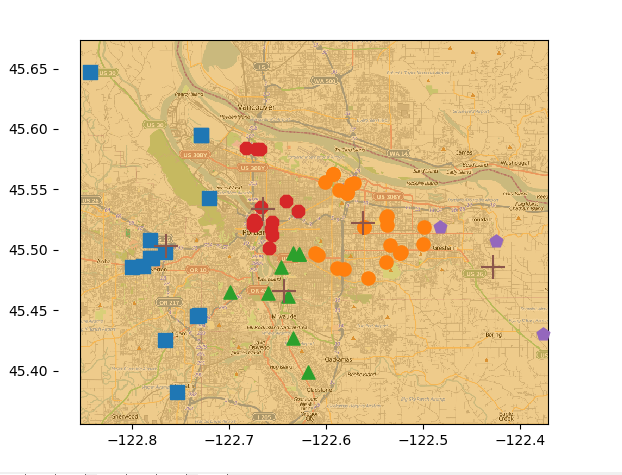k算法 机器学习实战 相关内容